• Introduction
• What is in this manual
• What is Caspoc
• User interface
• Introduction
• Starting
• Simulation
• Editing
• Viewing and printing
• Getting Started
• Basic editing
• Simulation in the time domain
• Basic User Interface Topics
• Editing
• Simulation
• Viewing
• Library
• Reports
• Project management
• Circuit and Block Diagram Components
• Introduction
• Cscript and user defined functions
• Component parameters
• Modeling Topics
• Introduction
• Power Electronics
• Semiconductors
• Electrical Machines
• Electrical drives
• Power Systems
• Mechanical Systems
• Thermal Systems
• Magnetic Circuits
• Green Energy
• Coupling to FEM
• Experimenter
• Analog hardware description language
• Embedded C code Export
• Coupling to Spice
• Small Signal Analysis
• Matlab coupling
• Tips and tricks
• Appendices

## Customized library drawings

For each library component you can create a customized drawing. The drawing is created using vector drawing commands. An overview of these commands is given in this section. Some examples of these customized drawings are given below.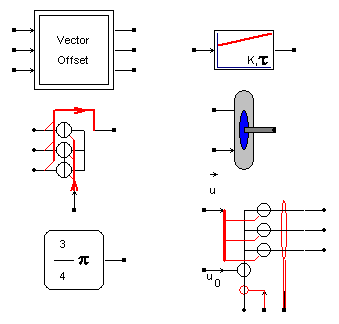To correctly display such a drawing the vector file should have the same file name as the library block, but with the *.vec extension. Example:

c:\MyLibrary\MyControlBlock\MyBlock.lib
c:\MyLibrary\MyControlBlock\MyBlock.vec
such a drawing, open the library properties dialog box by right mouse button clicking the library block.

To create such a drawing, open the library properties dialog box by right mouse button clicking the library block.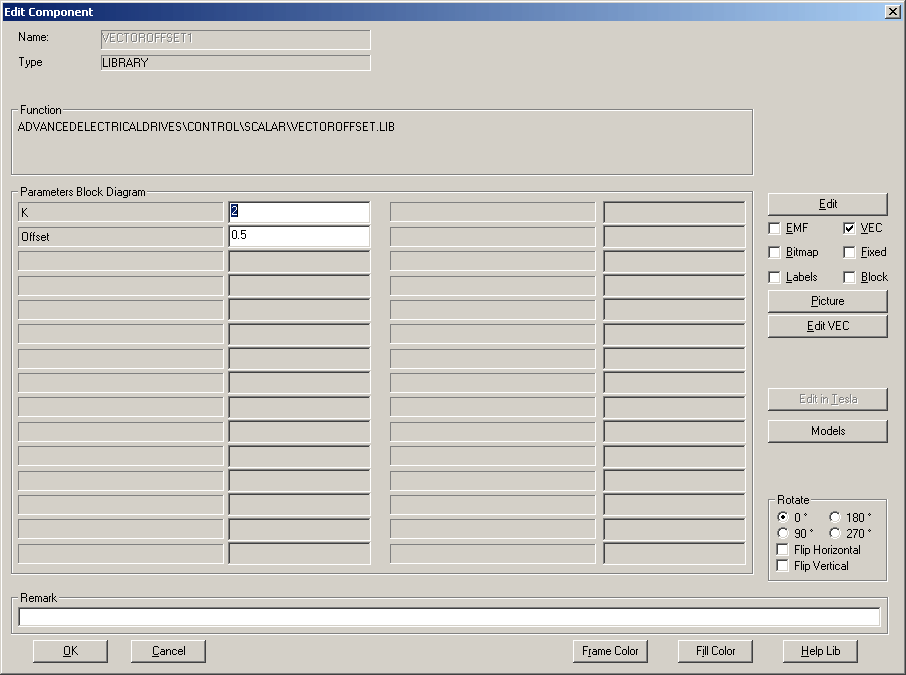Inside the properties dialog box you have to indicate that only the customized drawing should be displayed. Therefore check only the [VEC] box.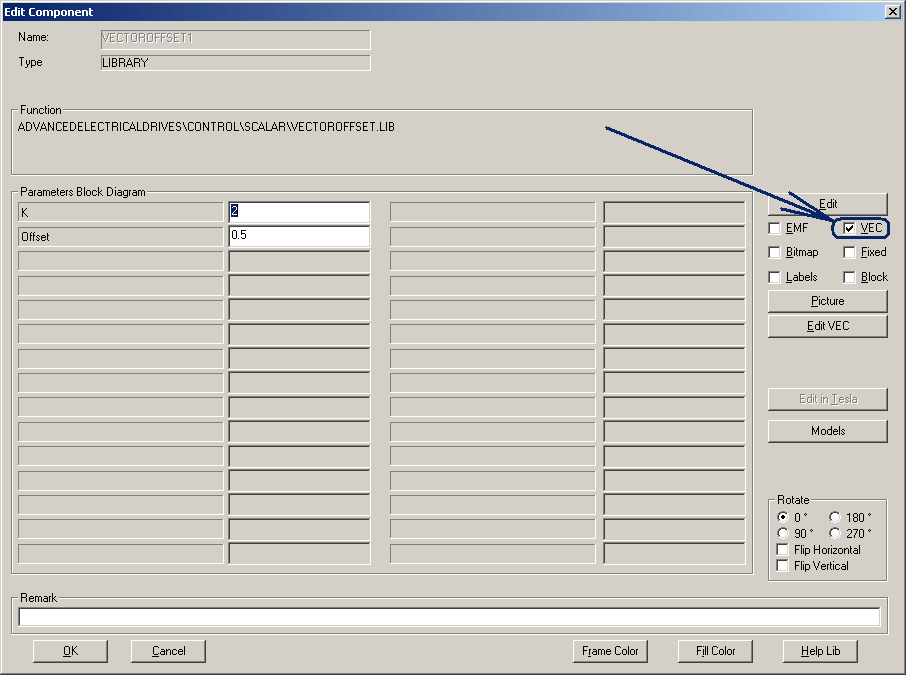To create a new drawing, click the [Edit VEC] button. A new *.vec block is created and you default text editor is opened with the *.vec file.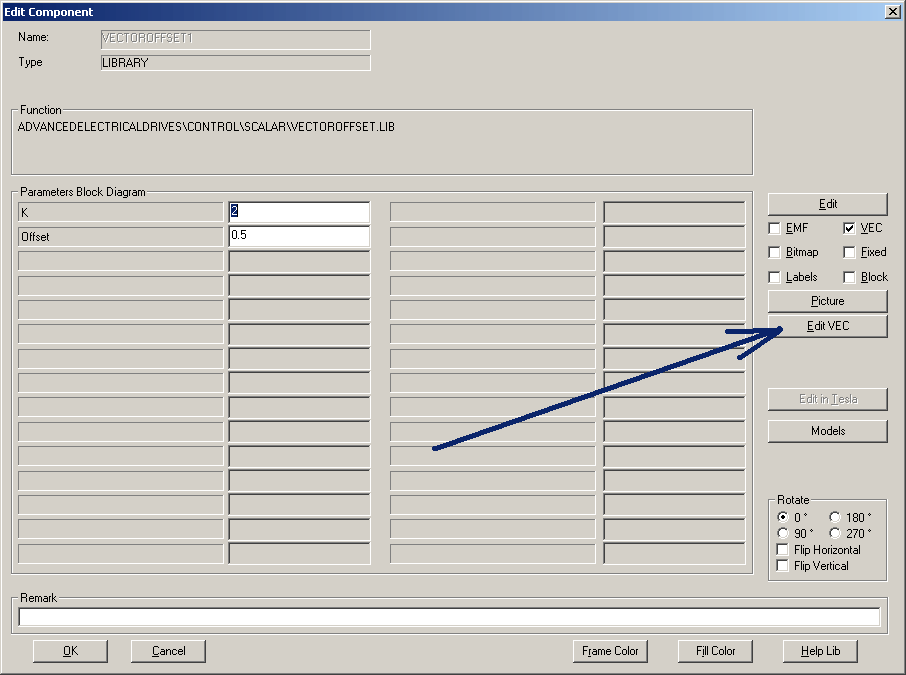Once the file is created, you can start typing commands in the vec file.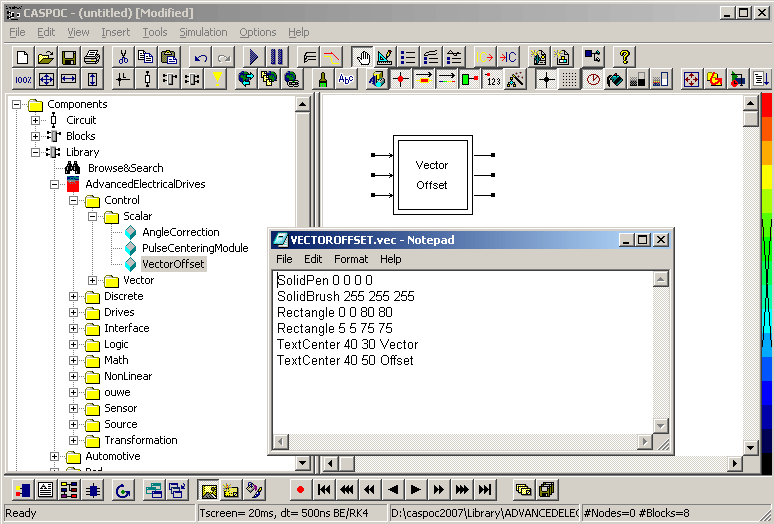In the example shown below a double square is drawn with white fill color and black single pixel pen width. The text is centered using two textcenter commands. Comments can be inserted either using a * or a // keyword.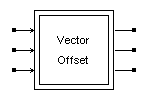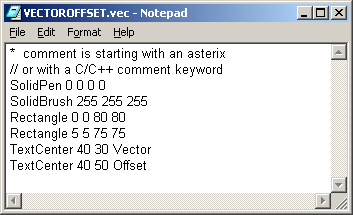The example shown above a single square is drawn with white fill color and black single pixel pen width. The red line is two pixels in width. The labels are centered using the label command.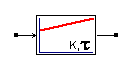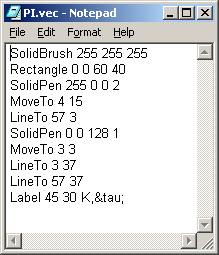## The drawing area

In Caspoc,a coordinate system is used that has its origin (0, 0) on the top-left corner of the drawing area.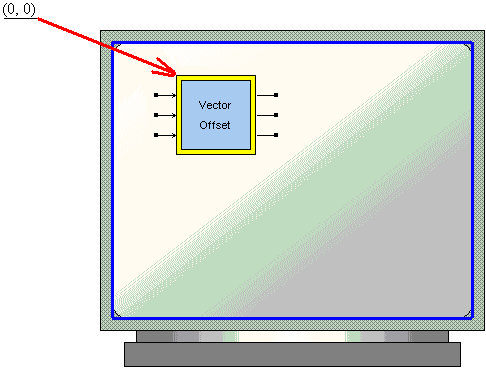Anything that is positioned on the screen is based on this origin. This coordinate system can get the location of an object using a horizontal and a vertical measurement. The horizontal measures are based on an x axis that moves from the origin to the right direction. The vertical measures use a y axis that moves from the origin to the bottom direction: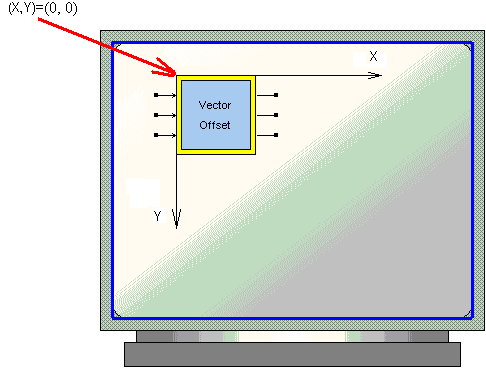This means that, if you start drawing something such as a line, it would start on the origin and continue where you want it to stop.

## SOLIDPEN

You can define the pen color as well as the thickness of the line. The pen color is define by the command

SolidPen R G B PenWidth
where R G B are the colors Red Green and Blue defined from 0 to 255 PenWidth is the width of the pen. You can specify the color by specifying a value for R, G and B from 0 to 255. 0 means no color, 255, means full intensity of the color. By mixing the RGB colors Red Green and Blue, 256*256*256 different colors can be generated.
The Penwidth varies from 0 to a larger value and defines the penwidth in pixels. By defining 0 for the penwidth, the pen is 1 pixel in width regardless of the size of he figure and the zoom factor. A penwidht of 3 is three pixels in width and will always display as thre times thicker than a penwidth of 1.
Examples of SolidPen are: Black line with minimum pen width:
Solidpen 0 0 0 0
Red line with minimum pen width:
Solidpen 255 0 0 0
Yellow Pen which is 3 pixels in width
SOLIDPEN 255 255 0 3
Black Pen which is 6 pixels in width
solidpen 0 0 0 0

## SOLIDBRUSH

You can define the background and fill by the command

Solidbrush R G B
where R G B are the colors Red Green and Blue defined from 0 to 255 You can specify the color by specifying a value for R, G and B from 0 to 255. 0 means no color, 255, means full intensity of the color. By mixing the RGB colors Red Green and Blue, 256*256*256 different colors can be generated.
All Rectangle, RoundRect, Ellipse and Pie commands are filled using the Solidbrush color.
Examples of SolidPen are:
White:
SOLIDBRUSH 255 255 255
Light gray:
SOLIDBRUSH 192 192 192
Dark gray:
SOLIDBRUSH 128 128 128
Red:
SOLIDBRUSH 255 0 0
Yellow:
SOLIDBRUSH 255 255 0

## HOLLOWBRUSH

Transparant:

HOLLOWBRUSH

## MOVETO

A line is a junction of two points. This means that a line has a beginning and an end: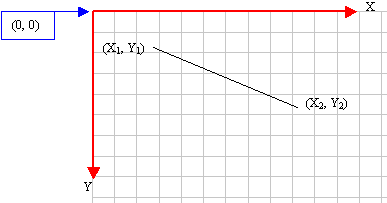The beginning and the end are two distinct points. In real life, before drawing, you should define where you would start. To help with this, the MoveTocommand can be used. Its syntax is:

MoveTo X Y

The X argument represents the horizontal distance of the line beginning from the (0, 0) origin.

The Y value is the vertical distance from the (0, 0) origin.
Here is an example that draws a line starting at a point defined as (20, 15)

	  MoveTo  20 15


## LINETO

To end the line, you use the LineTo command. Its syntax is:

LineTo  X  Y

The X argument represents the horizontal end of the line from the (0, 0) origin.

The Y value is the vertical end of the line from the (0, 0) origin.

Here is an example that draws a line starting at a point defined as (20, 15) coordinates and ending at (255, 82):



MoveTo  20 15
LineTo 255 82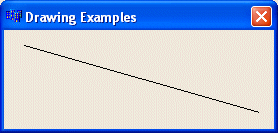We have mentioned that the MoveTo command is used to set the starting position of a line. When using LineTo, the line would start from the MoveTo point to the LineTo end. As long as you do not call MoveTo, any subsequent call to LineTo() would draw a line from the previous LineTo to the new LineTo point. You can use this property of the LineTo method to draw various lines. Here is an example:


MoveTo  60  20
LineTo  60 122
LineTo 264 122
LineTo  60 20



## POLYLINE

You can also use the statement Polyline to make a line.

	Polyline 60 20 60 122 264 122 60 20


## POLYLINETO

When you want to draw a line from your last point to a next point u can use the statement PolylineTo.
	MoveTo  10 10
LineTo  60 12
PolylineTo 100 50


## POLYBEZIER

Draws a bezier line from (x1,y1) to (x4,y4), controlled by two control points (x2,y2) and (x3,y3)

Polybezier x1 y1 x2 y2  x3 y3  x4 y4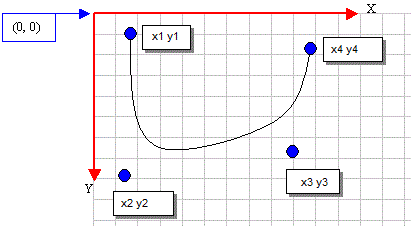## RECTANGLE

A rectangle is a geometric figure made of four sides that compose four right angles. Like the line, to draw a rectangle, you must define where it starts and where it ends. This can be illustrated as follows: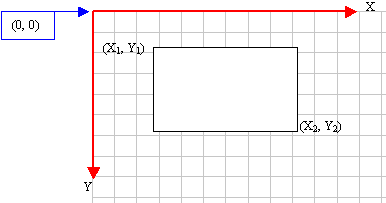The drawing of a rectangle typically starts from a point defined as (X1, Y1) and ends at another point (X2, Y2). To draw a rectangle, you can use the Rectangle command. Its syntax is:

Rectangle X1 Y1 X2  Y2

As seen on the figure and the formula, a rectangle spans from coordinates (x1, y1) to (x2, y2). Here is an example:



Rectangle 20  20  226 144



## ROUNDRECT

A rectangle qualifies as round if its corners do not form straight angles but rounded corners. It can be illustrated as follows: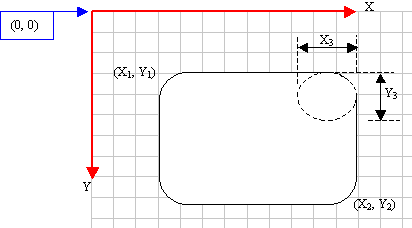To draw such a rectangle, you can use the RoundRect command. Its syntax is:

RoundRect  X1 Y1 X2 Y2 X3 Y3

When this member function executes, the rectangle is drawn from the (x1, y1) to the (x2, y2) points. The corners are rounded by an ellipse whose width would be x3 and the ellipse's height would be x3.

Here is an example:



RoundRect 20 20 275 188 42 38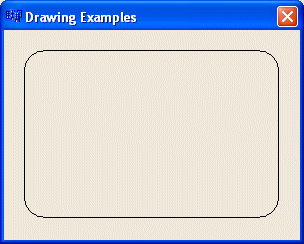A round square is a square whose corners are rounded.

## Polygon

This statement draws a polygon and fills it with the current brush. Using the following syntax:

Polygon x1 y1 x2 y2 x3 y3 .......  x1 y1


## PARALLELOGRAM

You can make a 3D block. First you specify a rectangle using the first four parameters. Next comes the depth, followed by the color specified by its R G B parameters. Using the following syntax:

Parallelogram x1 y1 x2 y2 z r g b


## Circle

A circle is easier to draw than using the ellipse command, since you only define the center of the circle and its radius.

	Circle xm ym R
which draws a circles at the center point (xm,ym) and radius R.

Here is an example:

	Circle 20 20 5


## ELLIPSE

An ellipse is a closed continuous line whose points are positioned so that two points exactly opposite each other have the exact same distant from a point called the center. It can be illustrated as follows: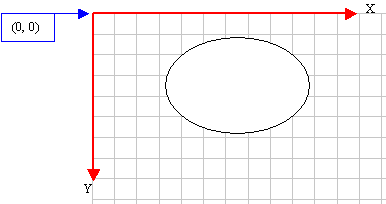Because an ellipse can fit in a rectangle, in GDI programming, an ellipse is defined with regards to a rectangle it would fit in. Therefore, to draw an ellipse, you specify its rectangular corners. The syntax used to do this is:

Ellipse X1 Y1 X2 Y2

The arguments of this method play the same roll as those of the Rectangle() method: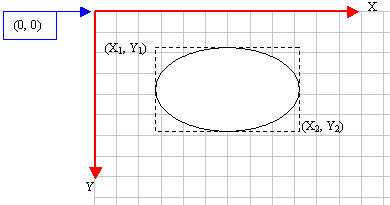Here is an example:



Ellipse 20 20 226 144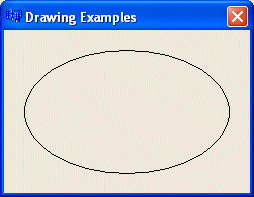A circle is an ellipse whose all points have the same distance with regards to a central point.

## Chords

The arcs we have drawn so far are considered open figures because they are made of a line that has a beginning and an end (unlike a circle or a rectangle that do not). A chord is an arc whose two ends are connected by a straight line. In other words, a chord is an ellipse that is divided by a straight line from one side to another: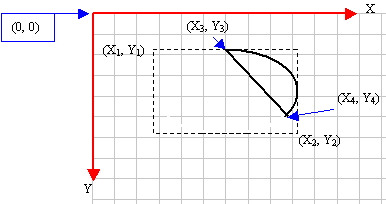To draw a chord, you can use the Chord() method. It is defined as follows:

Chord x1 y1 x2 y2 x3 y3 x4 y4


The x1, y1, x2, and y2 are the coordinates of the rectangle in which the chord of the circle would fit. These x3 and y3 coordinates specify where the arc that holds the chord starts. The x4 and y4 that can also be defined as ptEnd specify the end of the arc. To complete the chord, a line is drawn from (x3, y3) to (x4, y4). Here is an example:

Chord(20, 20, 226, 144, 202, 115, 105, 32)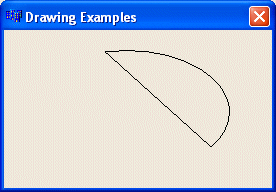## PIE

A pie is a fraction of an ellipse delimited by two lines that span from the center of the ellipse to one side each. It can be illustrated as follows: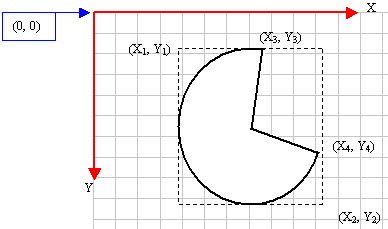To draw a pie, you can use the Pie method whose syntax is:

Pie X1 Y1 X2 Y2 X3 Y3 X4 Y4

The (X1, Y1) point determines the upper-left corner of the rectangle in which the ellipse that represents the pie fits. The (X2, Y2) point is the bottom-right corner of the rectangle.

The (X3, Y3) point specifies the starting corner of the pie in a default counterclockwise direction.

The (X4, Y4) point species the end point of the pie.

To complete the pie, a line is drawn from (X3, Y3) to the center and from the center to the (X4, Y4) points.

Here is an example:



Pie 40 20 226 144 155 32 202 115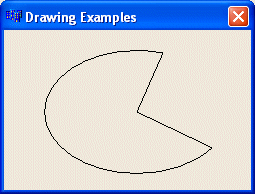## ARC

An arc is a portion or segment of an ellipse, meaning an arc is a non-complete ellipse. Because an arc must confirm to the shape of an ellipse, it is defined as it fits in a rectangle and can be illustrated as follows: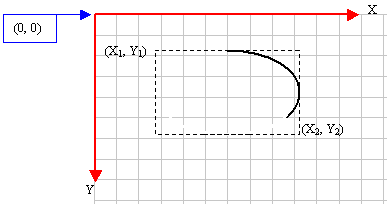To draw an arc, you can use the Arc method whose syntax is:

Arc x1 y1 x2 y2 x3 y3 x4 y4


Besides the left (x1, y1) and the right (x2, y2) borders of the rectangle in which the arc would fit, an arc must specify where it starts and where it ends. These additional points are set as the (x3, y3) and (x4, y4) points of the figure. Based on this, the above arc can be illustrated as follows: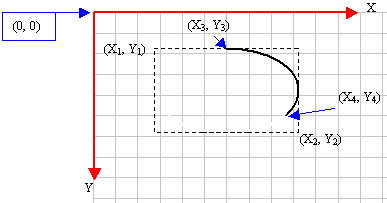Here is an example:

Arc(20, 20, 226, 144, 202, 115, 105, 32)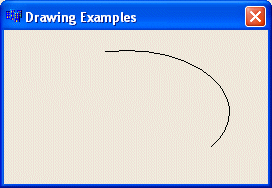The default value of the direction is AD_COUNTERCLOCKWISE.

## ARCTO

The ArcTo function is the same as the Arc function, only a line is drawn from the current position to the beginning of the arc.

## ANGLEARC

You can (also) draw an arc using the AngleArc() method. Its syntax is:

AngleArc x y Radius StartAngle SweepAngle


This member function draws a line and an arc connected. The arc is based on a circle and not an ellipse. This implies that the arc fits inside a square and not a rectangle. The circle that would be the base of the arc is defined by its center located at C(x, y) with a radius of Radius. The arc starts at an angle of StartAngle. The angle is based on the x axis and must be positive. That is, it must range from 0° to 360°. If you want to specify an angle that is below the x axis, such as -15°, use 360º-15°=345°. The last argument, SweepAngle, is the angular area covered by the arc. The AngleArc() method does control where it starts drawing. Here is an example:

AngleArc 100 100  50 0 90


centre is 100, 100, the radius equals 50 and it startss at 0 degrees and sweeps 90 degrees counter clock wise.

## Arrow

An arrow is specified using a starting and ending point Its syntax is:

Arrow xbegin ybegin xend yend


## ARCARROW

The ArcArrow is defined by its center, radius and angular position Its syntax is:

ArcArrow x y radius angle


## ARROWDOUBLE

An arrowdobble is specified using a starting and ending point Its syntax is:

ArrowDouble xbegin ybegin xend yend


## ANGLEARCARROW

Angular arrows are specified the same way as the angular arcs. Its syntax is:

AngleArcArrow x y Radius StartAngle SweepAngle


## TEXTOUT

To write text, you can call the TextOut command. Its syntax is:

TextOut X Y Text

The TextOut command is used to create an display a piece of text on the screen. The X and Y arguments are the point coordinates of the top-left corner of the string being displayed. The Text argument is the text that needs to be displayed. Here is an example:


TextOut 10 10 Vector Control Block


## TEXTCENTER

To write centered text, you can call the TextCenter command. Its syntax is:

TextCenter X Y Text

The TextCenter command is used to create and display a piece of text on the screen. The X and Y arguments are the center point coordinates of the string being displayed. The Text argument is the text that needs to be displayed. Here is an example:


TextCENTER 20 30 PI


## DRAWTEXTCENTER

To write a centered textblock, you can call the DrawTextCenter command. Its syntax is:

DrawTextCenter X1 Y1 X2 Y2 Text

The DrawTextCenter command is used to create and display a text block on the screen, where the text is truncated within the rectangle defined by X1,Y1 as left top corner and X2,Y2 as right bottom corner. The Text argument is the text that needs to be displayed. Here is an example:


DrawTextCenter 20 30 40 60 A long line of text that is truncated to fit into the rectangle defined by x1,y1 and x2,y2


## DRAWTEXTLEFT

To write a left aligned centered textblock, you can call the DrawTextLeft command. Its syntax is:

DrawTextLeft X1 Y1 X2 Y2 Text

The DrawTextLeft command is used to create and display a left aligned text block on the screen, where the text is truncated within the rectangle defined by X1,Y1 as left top corner and X2,Y2 as right bottom corner. The Text argument is the text that needs to be displayed. Here is an example:


DrawTextLeft 20 30 40 60 A long line of left aligned text that is truncated to fit into the rectangle defined by x1,y1 and x2,y2


## DRAWTEXTRIGHT

To write a right aligned centered textblock, you can call the DrawTextRight command. Its syntax is:

DrawTextRight X1 Y1 X2 Y2 Text

The DrawTextRight command is used to create and display a right aligned text block on the screen, where the text is truncated within the rectangle defined by X1,Y1 as right top corner and X2,Y2 as right bottom corner. The Text argument is the text that needs to be displayed. Here is an example:


DrawTextRight 20 30 40 60 A long line of right aligned text that is truncated to fit into the rectangle defined by x1,y1 and x2,y2


## LABEL

To write a label with Greek and mathematical characters, you can call the Label command. Its syntax is:

Label X Y Text

The Label command is used to create an display a piece of text on the screen. The X and Y arguments are the center point coordinates of the label being displayed. The Text argument is the text that needs to be displayed and may not contain any spaces. Here is an example:


Label 20 30 &Psi;_R

that draws the label ΨR

## LabelPlusMin

To write a label with a plus + and minus - sign to indicate a voltage level, you can call the LabelPlusMin command. Its syntax is:

LabelPlusMin X Y Text Angle[Optional] Height[Optional]

The LabelPlusMin command is used to create and display a piece of text on the screen. The X and Y arguments are the center point coordinates of the label being displayed. The Text argument is the text that needs to be displayed and may not contain any spaces. The Angle is optional and initially set to 90 degrees. The Height is optional and initially set to 20 pixels. This means that the + and - sign are displayed with a distance of Height pixels. Here is an example:

	LabelPlusMin 20 30 V_dc
LabelPlusMin 20 30 V_emf 180 40

that draws the label + Vdc - aligned vertically and the label + Vemf - aligned horizontally.

## LabelCurrent

To write a label with an arrow pointer to indicate a current flow direction, you can call the LabelCurrent command. Its syntax is:

LabelCurrent X Y Text Angle[Optional]

The LabelCurrent command is used to create and display a current flow. The X and Y arguments are the center point coordinates of the arrow pointer being displayed. The Text argument is the text that needs to be displayed and may not contain any spaces. The Angle is optional and initially set to 0 degrees. Here is an example:

	LabelCurrent 20 30 I_dc
Labelcurrent 20 30 I_out 270

that draws the label Idc above an arrow pointer from left to right and the label Iout aligned left to an arrow pointer pointing downwards.

## LabelArrow

To write a label with an arrow to indicate a current flow direction, you can call the LabelArrow command. Its syntax is:

LabelArrow Xb Yb Xe Xe Text

The LabelArrow command is used to create and display a current flow. The Xb,Yb and Xe,Ye arguments are the beginning and end point coordinates of the arrow being displayed. The Text argument is the text that needs to be displayed and may not contain any spaces. Here is an example:

	LabelArrow 20 30 60 30 I_dc
Labelarrow 20 30 20 60 I_out

that draws the label Idc above an arrow pointer from left to right and the label Iout aligned left to an arrow pointer pointing downwards.

## LabelTo

To write a label with an arrow to indicate a specific point, you can call the LabelTo command. Its syntax is:

LabelTo Xb Yb Xe Xe Text

The LabelTo command is used to create and display a label with pointing arrow. The Xb,Yb and Xe,Ye arguments are the beginning and end point coordinates of the arrow being displayed. The Text argument is the text that needs to be displayed and may not contain any spaces. Here is an example:

	LabelTo 20 30 60 30 I_dc
Labelto 120 30 120 60 I_out

that draws the label Idc at the begin of the arrow pointer from left to right and the label Iout at the begin of an arrow pointer pointing downwards.

## TextTo

To write a Text with an arrow to indicate a specific point, you can call the TextTo command. Its syntax is:

TextTo Xb Yb Xe Xe Text

The TextTo command is used to create and display a text with pointing arrow. The Xb,Yb and Xe,Ye arguments are the beginning and end point coordinates of the arrow being displayed. The Text argument is the text that needs to be displayed and may contain spaces, but odes not process greek characters neither sub- nor superscript. Here is an example:

	TextTo 20 30 60 30 Pointing to location(60,30)
textto 120 30 120 Another arrow pointing to a place on the screen

that draws the text at the begin of the arrow pointer from left to right and the second text at the begin of an arrow pointer pointing downwards.

## COMPONENT

You can draw any circuit component at a specific center point with the orientation defined by angle(0,90,180,270). If the last parameter angle is omitted, the component is drawn vertically. (default angle=90)

	Component R xm ym angle

The following electric circuit symbols can be drawn:
R, C, L, LBR, V, I, VAC, IAC, Vsquare, Vtriangle, A, B, E, F, G, H, D, MOSFET, nMOSFET, pMOSFET, IGBT, NPN, PNP, Zener, Schottky, S, SCR, GTO, Diac, Triac.

Example of an RC series circuit:

	Component R 60 0 0
Component c 120 0 0

Example of an RC parallel circuit:
	Component R 60 30
Component C 100 30
Polyline 60 0  100 0
Polyline 60 60  100 60


## BLOCK

Draw a block diagram component

	Block type xm ym
Example:
	Block Xor 20 20

## SYMBOL

Draw a symbol

	Symbol type xm ym angle H/V
where xm,ym is the position ofh te symbol, angle(=0, 90,180 or 270) the orientation and H or V specifies if the symbol has to be mirrored along a Vertical or Horizontal axis. Example:
	Symbol Xor 20 20
	Symbol Latch 120 40 90 V
	Symbol not 40 20 270

• NPN
• PNP
• AND
• OR
• XOR
• NAND
• NOT
• BUFFER
• COMP
• COMPSMALL
• LATCH
• GROUND

## OBJECT

All animation objects from the block diagram block OBJECT can be drawn using the following syntax:

Object p1 i1 i2 i3 i4 i5 i6 i7 i8 i9 i10 i11 i12 i13 i14 i15 i16 i17 i18 i19 i20


	Object type  xm ym w h
Example:
Rectangle(type=10) positioned from (20,40) to (20+5,40+10):
	Object 10  20 40 5 10

## DIP

Draws a Dual In-line Package from x1,y1 to x2,y2

	DIP x1 y1 x2 y2 [w]
The distance between the pins is defined by the optional parameter w which defaults to 20.

## PDIP

Draws a Plastic Dual In-line Package

	PDIP pins
The parameter pins defines the total number of pins. Example:
8 pins package for a single opamp
	pdip 8

## ICPIN

Draws a labeled IC pin onto an IC package.

	ICpin x y label L/R/T/B
The label is displayed within the ICpin positioned t x,y. The parameter L/R/T/B defines whether the label is positioned left, right, top or bottom aligned to the package. Example:
Two pins on the left sie and 2 pins on the right side
	ICpin 0 10 1 L
	ICpin 0 30 2 L
	ICpin 60 10 4 R
	ICpin 60 30 3 R

## OFFSET

Offsets the cursor and regards this position as 0,0. Using the following syntax:

Offset x y